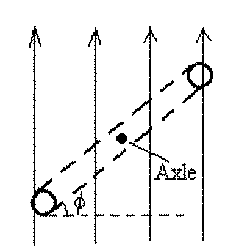## University of California, San Diego Physics 1b - Thermal Physics & Electromagnetism

 H. E. Smith Spring 2000Physics 1B - Tutorial #91. The figure above shows two long current-carrying wires. What is the value of the magnetic field at point P, 1m away from the crossing point? What would be the value of the magnetic field if one of the currents were reversed?

2. The figure (Hecht p. 820, q. 4) shows an electric door bell. The circuit consists of a battery, a switch at the door, a "make-break" contact and an electromagnet. Explain what happens when the switch is pressed. Is this a "ding-dong" chime, a buzzer, or what?3. The figure shows several different loops in magnetic fields. In each case the magnetic field is perpendicular to the plane of the loop and the field strength is indicated/ The numbers by the sides of the loops indicate the lengths of the sides. Order the loops in order of magnetic flux, from smallest to largest.4. A circular conducting loop rotates at constant angular speed about an axle through the center of the loop. The figure shows an edge-on view and defines an angle which increases from 0 to 360° as the loop rotates.
• At what angle(s) is the magnetic flux a maximum?
• At what angle(s) is the magnetic flux a minimum?
• At what angle(s) is the magnetic flux changing most rapidly?
• At what angle(s) is the induced emf a maximum?Physics 1B HomeTutorials

Gene Smith